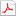검색어 입력폼

# [전기전자전파] 전기회로 5장 연습문제풀이

저작시기 2009.04 |등록일 2009.04.21어도비 PDF (pdf) | 21페이지 | 가격 2,000원

전기회로 5장 연습문제풀이

없음

## 본문내용

5.1 The equivalent model of a certain op amp is shown in Fig. 5.43.Determine:

5.5 For the op amp circuit of Fig. 5.44, the op amp has an open-loop gainof 100,000, an input resistance of 10kΩ. Find the voltage gain using the nonidealmodel of the op amp.

5.9 Determine for each of the op amp circuits in Fig. 5.48.

5.13 Find and in the circuit of Fig. 5.22.

5.15 (a) Determine the ratio in the op amp circuit of Fig. 5.54.

5.19 Determine in the circuit of Fig. 5.58.

5.23 For the op amp circuit in Fig. 5.61, find the voltage gain .

5.25 Calculate in the op amp circuit of Fig. 5.63.

5.29 Determine the voltage gain of the op amp circuit in Fig. 5.67.

5.33 Refer to the op amp circuit in Fig. 5.71. Calculate and the powerdissipated by the 3-kΩresistor.

5.37 Determine the output of the summing amplifier in Fig. 5.74.

5.41 An averaging amplifieris a summer that provides an output equal tothe average of the inputs. By using proper input and feedback resistorvalues, one can getUsing a feedback resistor of 10kΩ, design an averaging amplifier withfour inputs.

없음